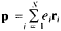# Dipole Moment, Electrical

The following article is from The Great Soviet Encyclopedia (1979). It might be outdated or ideologically biased.

## Dipole Moment, Electrical

a quantity used in physics to characterize the electrical properties of a system of charged particles. The dipole moment of a system consisting of N charged particles is, where e1 is the charge of a particle of the i number and r1 is its radius vector. The dipole moment of a charge system that is neutral as a whole does not depend on the choice of origin for coordinates but is determined by the relative location (and the magnitude) of the charges in the system. In a special case, a neutral system consisting of two charges (+e and −e} forms an electric dipole having a dipole moment p = eI, where I is the radius vector plotted from the negative charge to the positive charge. In the case of an arbitrary system of charged particles, its electric field at a great distance from the system is equal to the sum of various multiple fields, which are determined by complete charge, dipole moment, or quadrupole moment. However, if the distance from the system is great in comparison with the system’s dimensions, the electric field of a neutral system is defined, in the first approximation, solely by its dipole moment. Radiation of electromagnetic waves influenced by a time-dependent change of the dipole moment of the system is called dipole radiation.

G. IA. MIAKISHEV